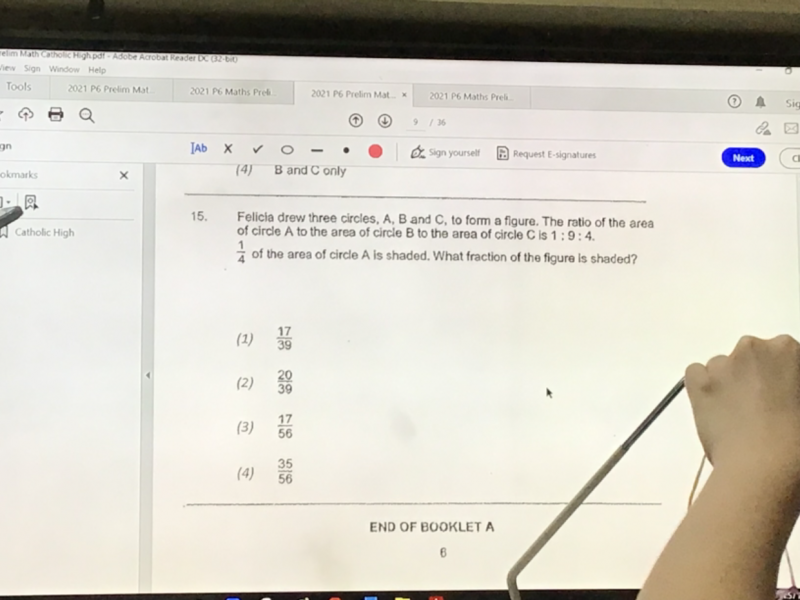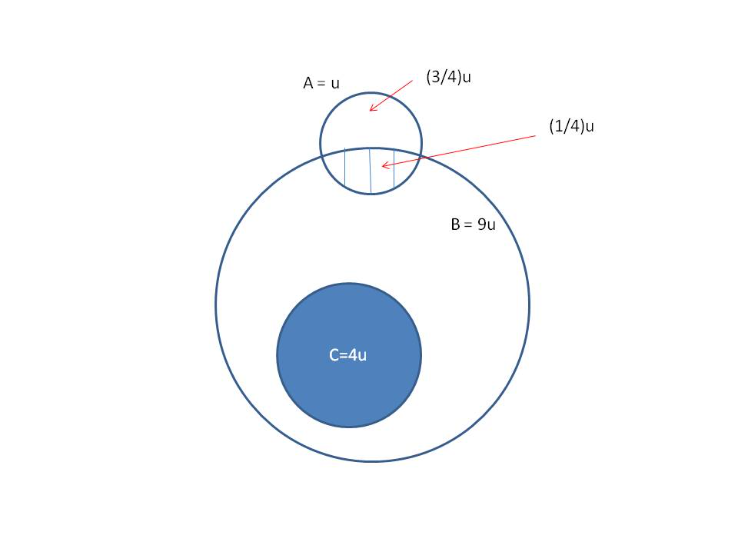# QuestionPls helpHi Aaron,

This question is missing a picture and hence it cannot be solved.  However, I found the picture to this question and here is my solution:

Total figure = 9u + (3/4) u = (39/4)u

Shaded part of figure = (1/4u) + 4u = (17/4)u

Hence fraction of the shaded part over the total figure

=       (17/4) u

————

(39/4) u

= 17/39

Hence the answer is (1) 17/39

0 Replies 1 Like ✔Accepted Answer